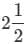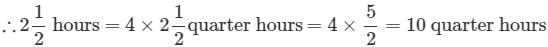# Test: Time - 1

## 10 Questions MCQ Test Mathematics for Class 4: NCERT | Test: Time - 1

Description
Attempt Test: Time - 1 | 10 questions in 20 minutes | Mock test for Class 4 preparation | Free important questions MCQ to study Mathematics for Class 4: NCERT for Class 4 Exam | Download free PDF with solutions
QUESTION: 1

### How many minutes are there in 2 hrs 30 minutes?

Solution:

In one hour = 60 minutes
∴ In 2 hours = 2 x 60 = 120

2 hours 30 minutes = 2 × 60 + 30 minutes
= 150 minutes

QUESTION: 2

### How many hours make 240 minutes?

Solution:

1 hour = 60 minutes

so 1 min = 1/60 hr

240 min = 240 x 1/60 = 4 hours

QUESTION: 3

### What is the time corresponding to p.m. in the 24 hour clock?

Solution:

Explanation:- 12 hrs is the am pm clock that time corresponding to p.m. in the 24 hour clock.

QUESTION: 4

What is the difference between 7 hours 25 minutes and 3 hours 45 minutes?

Solution:

7 hours 25 mins - 3 hours 45 mins = 3 hours 40 mins

QUESTION: 5

Leena will be 53 years old in 2019. What was her age in 1998?

Solution:

Difference between 2019 & 1998 is 21

53 - 21 = 32

QUESTION: 6

How many quarter minutes does the second hand of a clock complete to travel through from 6:15 p.m. to 6:18 p.m.?

Solution:

The correct option is Option C.

We know,

One quarter minute = 15 seconds

Now, the time difference between 6:15 p.m. and 6:18 p.m. is = 3 minutes

So, One whole minute has 60 seconds, which means

= 60 / 15 = 4 quarters

So, 3 minutes will have = 4 × 3 = 12 quarter minutes.

QUESTION: 7

How many quarter hours are there inhours?

Solution:

1 hour = 4 quarter hoursQUESTION: 8

The time is exactly 16:30 hours now. What time will it be after 120 seconds?

Solution:

60 seconds = 1 minute 120 second = 120/60 = 2 minutes .
∴ The time 120 seconds after 16:30 hours = 16:32 hours

QUESTION: 9

How is the time from 12 midnight to 12 noon denoted?

Solution:

The time from 12 midnight to 12 noon is the forenoon or the 1st half of the day denoted as a.m.

QUESTION: 10

Sudha works 6 hours 30 minutes each day how long will she work over 5 days?

Solution:

No. of hours of work per day = 6 hours 30 minutes No. of hours of work for 5 days = 6 hours 30 minutes × 5 = 30 hours 150 minutes 60 minutes = 1 hour
∴ 150 minutes = 2 hours 30 minutes
∴ 30 hours 150 minutes = 30 hours + 2 hours 30 minutes = 32 hours 30 minutesUse Code STAYHOME200 and get INR 200 additional OFF Use Coupon Code